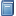Buchempfehlung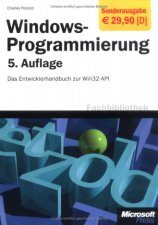Windows-Programmierung. Das Entwicklerhandbuch zur WIN32-API
"Der" Petzold, das über 1000 Seiten starke Standardwerk zum Win32-API - besonders nützlich u. a. bei der GUI-Programmierung in FreeBASIC! [Mehr Infos...]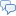FreeBASIC-Chat
Es sind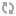Benutzer im FreeBASIC-Chat online.
(Stand:  )

Code-Beispiel

String-Array variabel zusammenführen

 Lizenz: Erster Autor: Letzte Bearbeitung: k. A.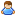ThePuppetMaster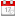17.10.2007
```declare Function F_CompareString(V_DD() as string, V_DC as long, V_SepString as string, V_StartItem as long, V_CountItem as Long) as string Dim DC as long Dim DD() as string DC = 5 Redim DD(DC) as string dd(1) = "abcd" dd(2) = "efgh" dd(3) = "ijkl" dd(4) = "mnop" dd(5) = "qrst" print "Alle Elemente zusammensetzen: "; F_CompareString(dd(),dc," ",1,0) print "2 elemente ab Element 3: "; F_CompareString(dd(),dc," ",3,2) print "Alle mit '_-_' füllen: "; F_CompareString(dd(),dc,"_-_",1,0)```

```Function F_CompareString(V_DD() as string, V_DC as long, V_SepString as string, V_StartItem as long, V_CountItem as Long) as string Dim X as long Dim X1 as long Dim X2 as long Dim D as string X1 = V_StartItem if X1 <= 0 then x1 = 1 X2 = X1 + V_CountItem - 1 if x2 < x1 then x2 = v_dc if X2 <= 0 then x2 = v_dc if X1 > V_DC then exit function if X2 > v_dc then X2 = V_DC for X = X1 to X2     D = D & V_DD(X) & V_SepString next if len(D) > 0 then D = mid(D,1,len(D)-len(V_SepString)) F_CompareString = D end function```
Zusätzliche Informationen und Funktionen
• Das Code-Beispiel wurde am 17.10.2007 vonThePuppetMaster angelegt.
• Die aktuellste Version wurde am 17.10.2007 vonThePuppetMaster gespeichert.